# SOCR EduMaterials Activities RandomVariableExperiment

## Description

The experiment simulates a value of a random variable with a specified distribution. The value is recorded on each update. The normal, gamma, chi-square, student t, F, beta, Weibull, Pareto, logistic, and lognormal distributions can be chosen from the list box. In each case, the parameters of the distribution can be varied with scroll bars. The density and moments of the distribution are shown in the distribution graph, and the moments are given in the distribution table.

## Goal

The main focus of this experiment is to give a simple simulation that demonstrates the distributions of random variables being selected under different types of conditions. After using this applet, users will be able to grasp a better understanding of the many types of distributions and be able to distinguish them easily.

## Experiment

Go to the SOCR Experiment and select the Random Variable Experiment from the drop-down list of experiments on the top left. The image below shows the initial view of this experiment: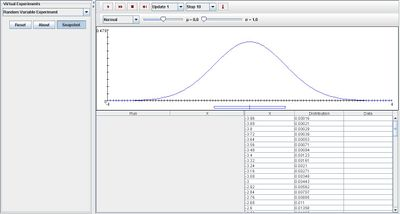When pressing the play button, one trial will be executed and recorded in the distribution table below. The fast forward button symbolizes the nth number of trials to be executed each time. The stop button ceases any activity and is helpful when the experimenter chooses “continuous,” indicating an infinite number of events. The fourth button will reset the entire experiment, deleting all previous information and data collected. The “update” scroll indicates nth number of trials (1, 10, 100, or 1000) performed when selecting the fast forward button and the “stop” scroll indicates the maximum number of trials in the experiment.

Normal

When selecting the normal distribution, varying the mean and standard deviation does not create a big impact on the outcome of the graphs. The empirical density graph will converge to the distribution graph as illustrated in the picture below: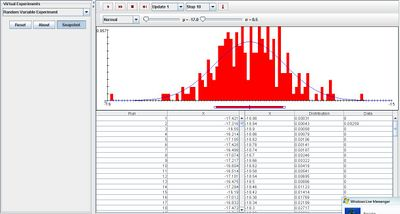Gamma

Under the gamma distribution, varying parameter b does not create a huge impact on the outcome of the experiment but modifying parameter k shifts the distribution graph. Initially, k has a small value so the distribution graph is purely skewed right, almost like a normal distribution curve centered at the vertical axis. Once k is increased, the graph is shifted to the right so that a peak is formed on the distribution graph. The image below demonstrates this as k obtains a large value – note that as the number of trials increase, the empirical density graph converges to the distribution graph: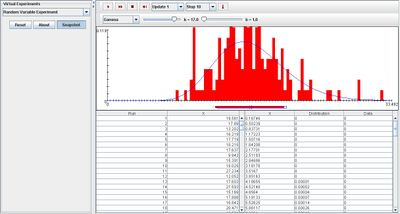Chi-square

For the Chi-square distribution, the distribution graph is initially skewed right. As parameter n increases, the graph shifts right and begins to take the shape of a normal curve. Even when n is at its maximum, it is not entirely normal but it may appear approximately normal. The image below illustrates this last statement: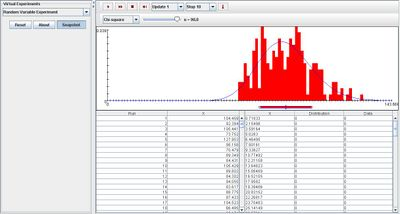Student

Varying parameter n of the student distribution has little effect on the distribution graph as increasing it will merely make the graph appear more normal: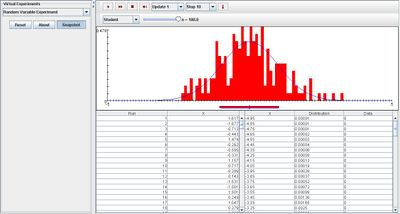F

For the F distribution, varying both parameters shifts the distribution graph to the right. At first, the graph is skewed right but as either one parameter or both parameters are increased, the distribution graph shifts right. The image shown below supports this: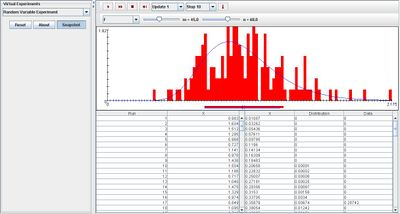Beta

For the beta distribution, varying parameters a and b change the distribution graph. Increasing parameter a will lower the severely decrease the left side of the distribution graph where most values are zero. Similarly, increasing parameter b will lower the right side of the distribution graph. The image shown below demonstrates this property with a large value of parameter a and b, in which the graph will appear normal: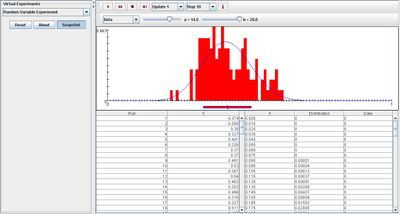Weibull

Increasing parameter b does not create a huge impact on the outcome of the experiment but parameter k does. When parameter k increases, the graph shifts to the right and beings to take form of a curve that is skewed left. The image below demonstrates this property: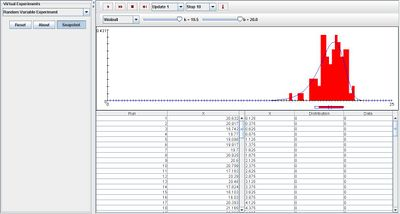Pareto

For the pareto distribution, varying parameter a has little effect on the outcome of the experiment. However, it does change the slope of the distribution graph slightly: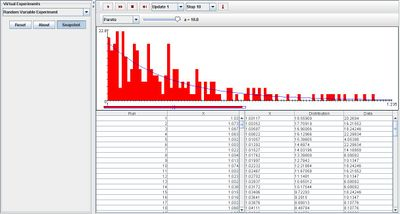Logistic

Take note that the empirical density graph converges to the distribution graph: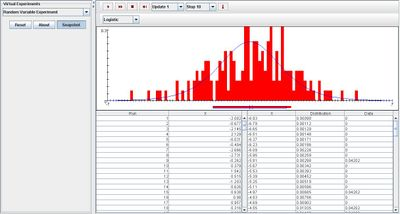Lognormal

Varying the mean does not change much of the distribution graph but varying the standard deviation does. Increasing the standard deviation will cause the distribution graph to collapse, thus when the standard deviation obtains a large value, the distribution graph will become extremely small and difficult to use as an illustration to present data information. The image shown below supports this statement: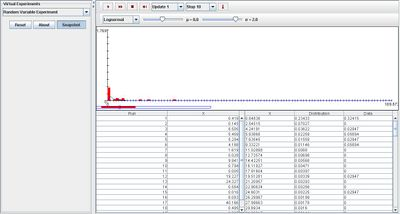Extreme Value

Take note that the empirical density graph converges to the distribution graph: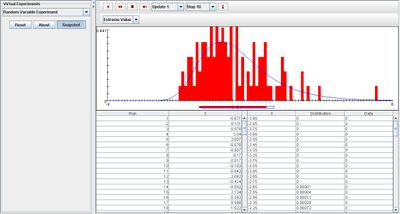## Applications

The Random Variable Experiment allows the public to explore the many basic distributions of probability and statistics. Not only could it be used to help others better understand and distinguish the many properties of each distribution, but it may be used in daily activities such as:

Suppose researchers want to illustrate their gathered data of the number of rainstorms per year over a course of ten years in a simple presentation. Using this applet and selecting a well-fit distribution will allow them to accomplish this task.

Suppose students need to explain their findings in a research lab. With this applet, they may select the appropriate distribution and select an approximate value for the parameters to help demonstrate their conclusion to their audience.